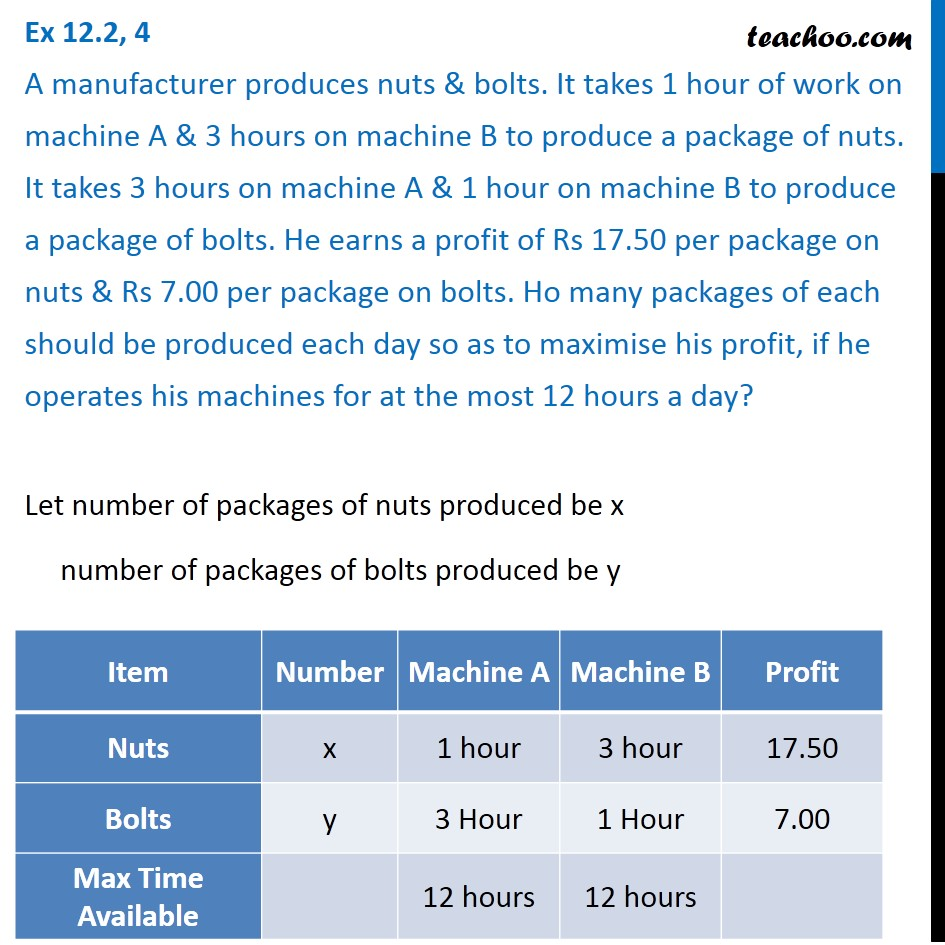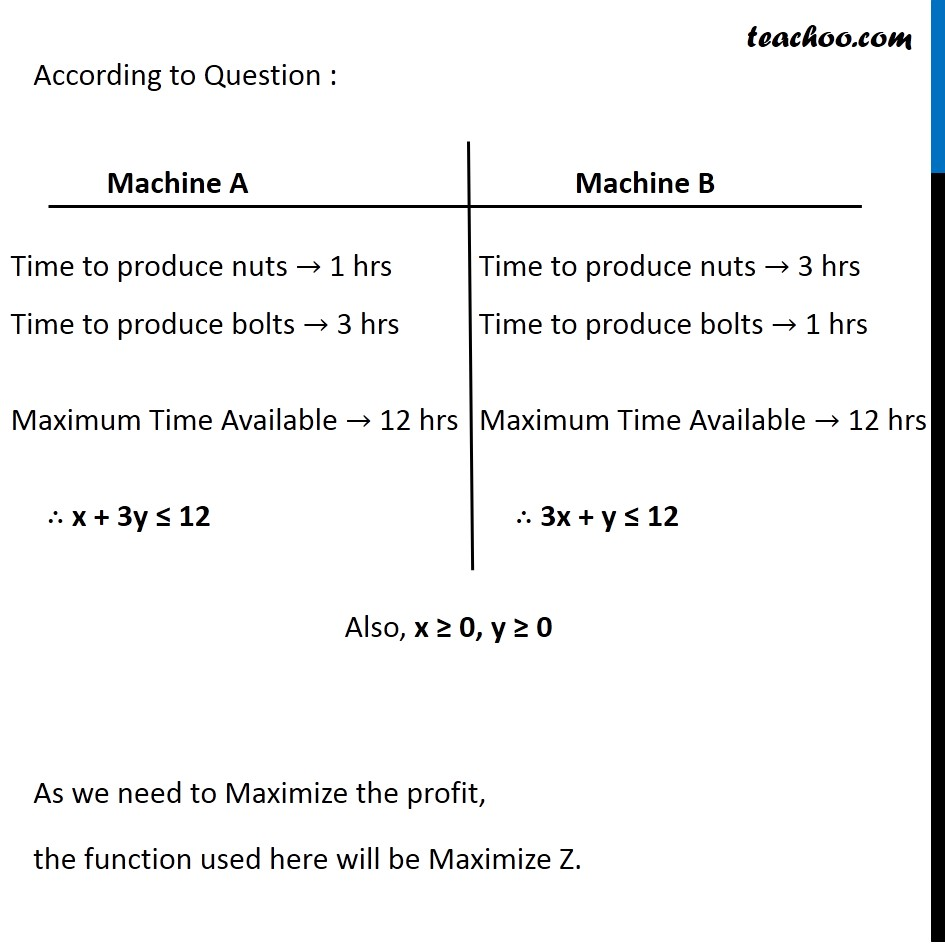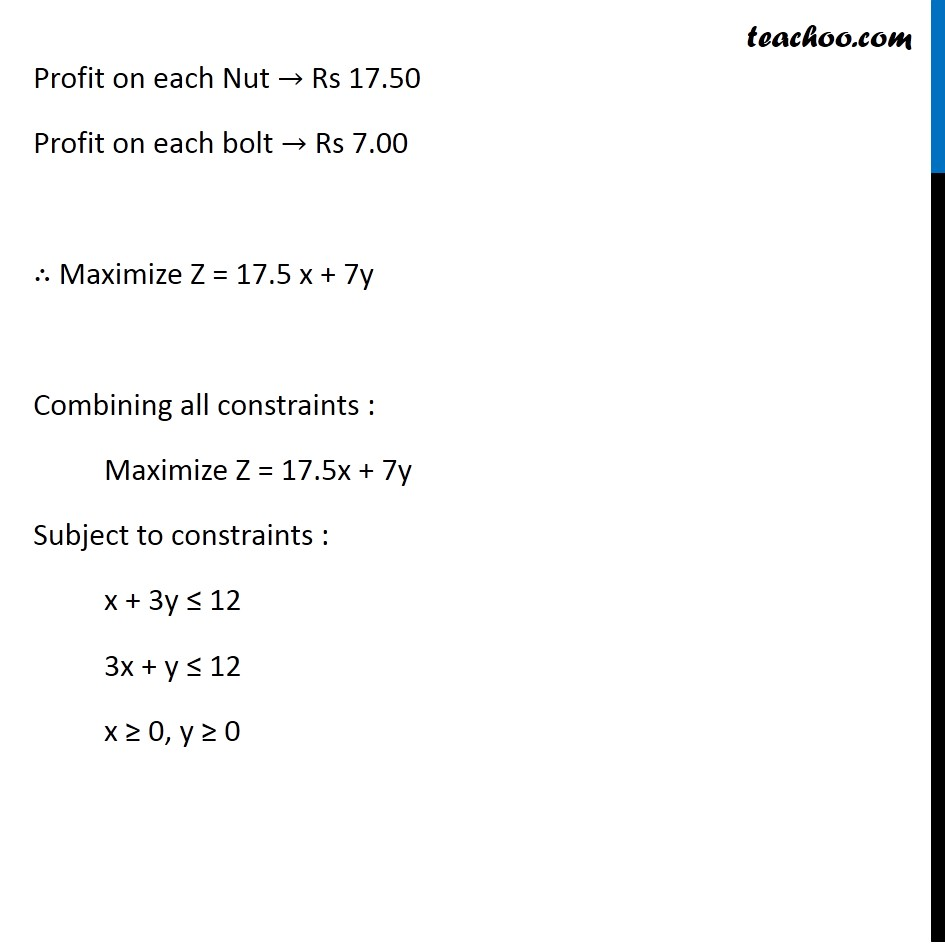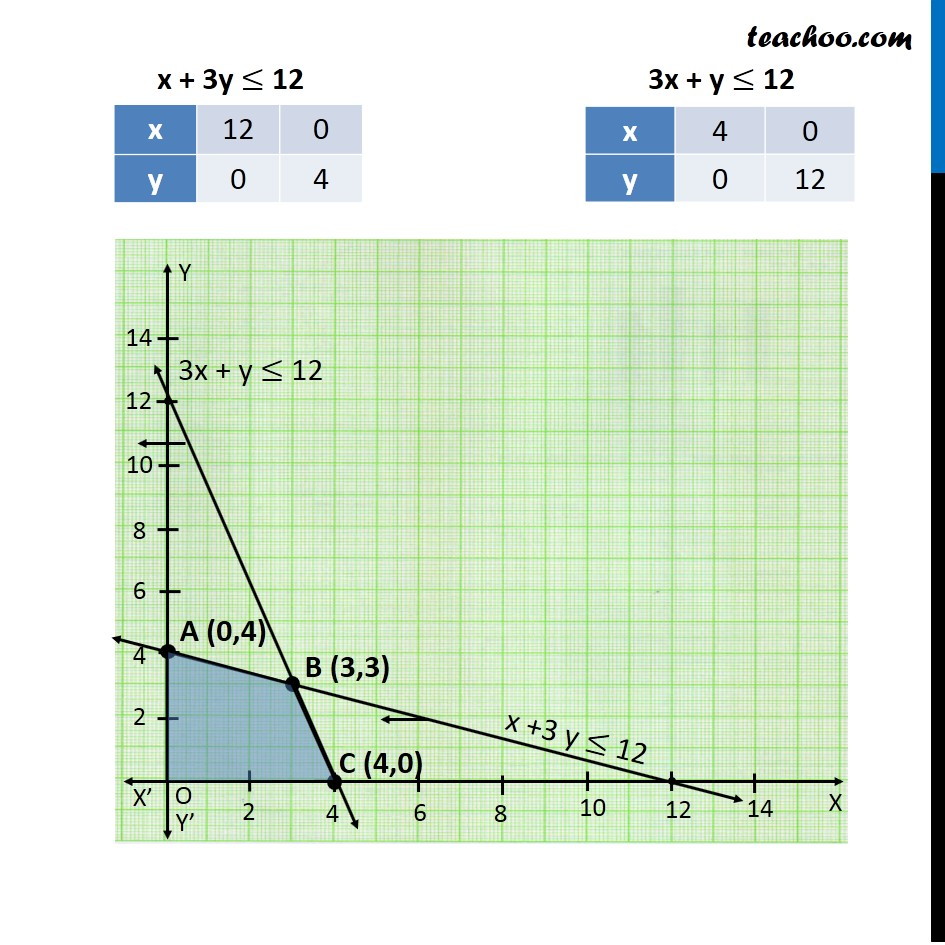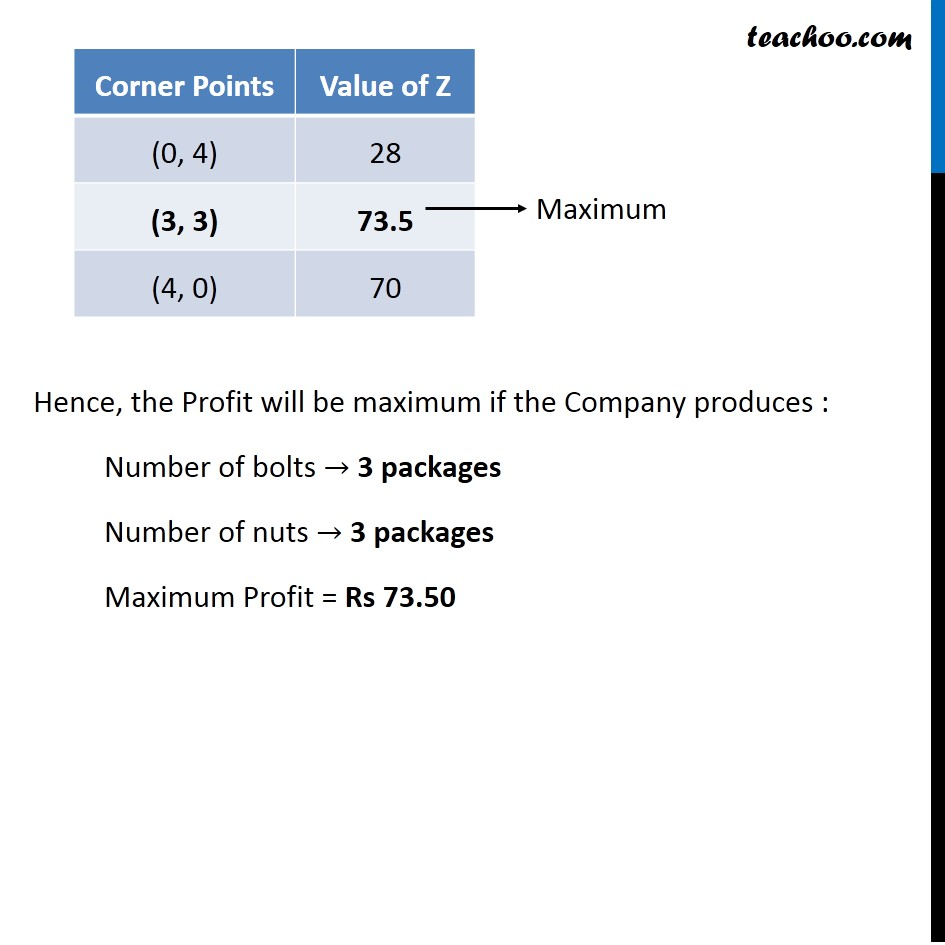1. Chapter 12 Class 12 Linear Programming (Term 1)
2. Serial order wise
3. Ex 12.2

Transcript

Ex 12.2, 4 A manufacturer produces nuts & bolts. It takes 1 hour of work on machine A & 3 hours on machine B to produce a package of nuts. It takes 3 hours on machine A & 1 hour on machine B to produce a package of bolts. He earns a profit of Rs 17.50 per package on nuts & Rs 7.00 per package on bolts. Ho many packages of each should be produced each day so as to maximise his profit, if he operates his machines for at the most 12 hours a day?Let number of packages of nuts produced be x number of packages of bolts produced be y According to Question : As we need to Maximize the profit, the function used here will be Maximize Z. Machine A Time to produce nuts → 1 hrs Time to produce bolts → 3 hrs Maximum Time Available → 12 hrs ∴ x + 3y ≤ 12 Machine B Time to produce nuts → 3 hrs Time to produce bolts → 1 hrs Maximum Time Available → 12 hrs ∴ 3x + y ≤ 12 Profit on each Nut → Rs 17.50 Profit on each bolt → Rs 7.00 ∴ Maximize Z = 17.5 x + 7y Combining all constraints : Maximize Z = 17.5x + 7y Subject to constraints : x + 3y ≤ 12 3x + y ≤ 12 x ≥ 0, y ≥ 0 Hence, the Profit will be maximum if the Company produces : Number of bolts → 3 packages Number of nuts → 3 packages Maximum Profit = Rs 73.50